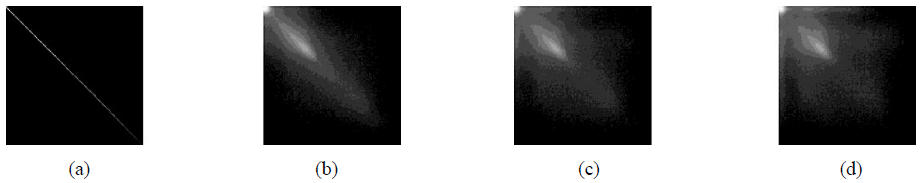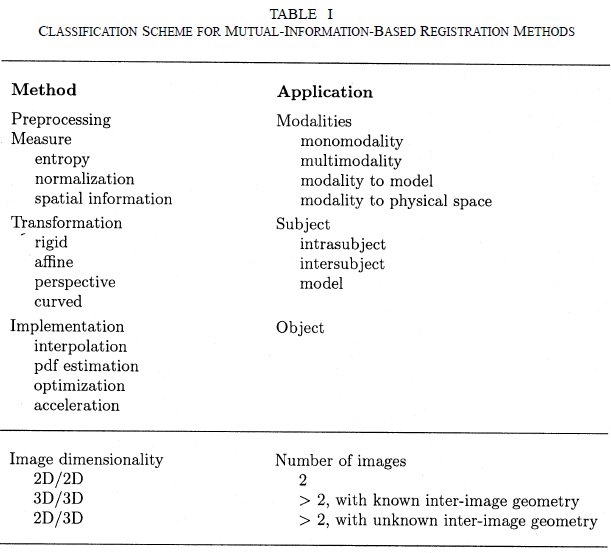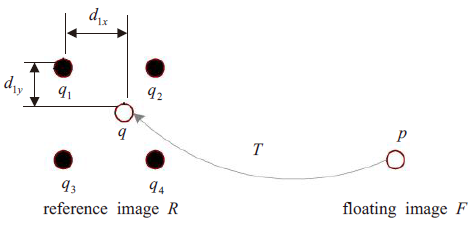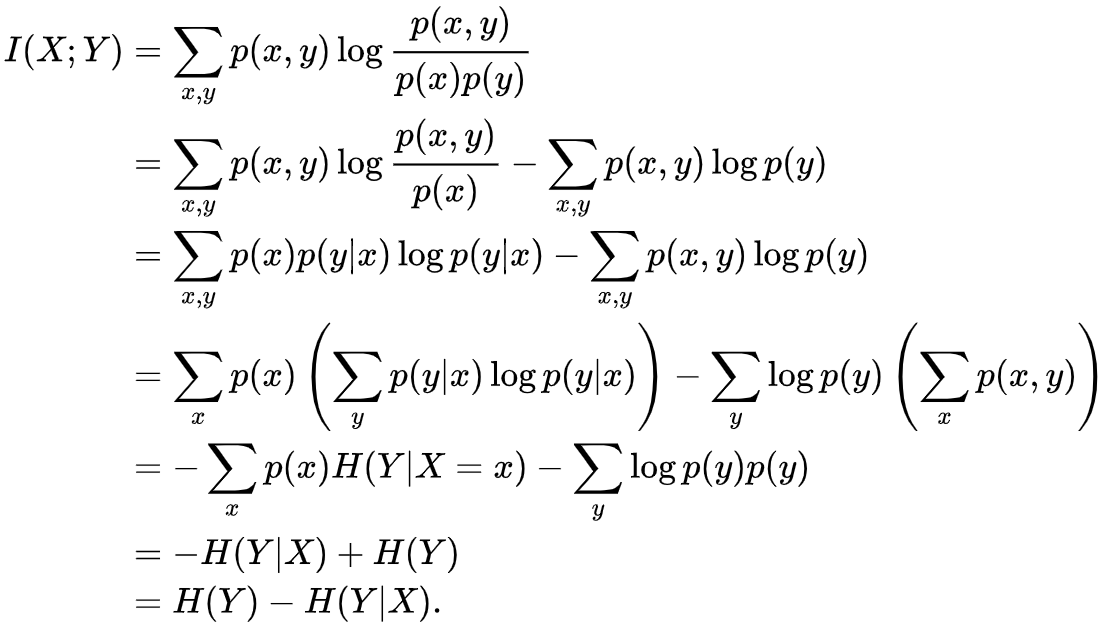# [论文笔记]基于互信息的医学图像配准综述

26 篇文章 2 订阅

2003年的综述类文章，目前已有3200+引用，经典文章，值得一读。

## 一、香农熵(Shannon entropy)

H = ∑ i p i log ⁡ 1 p i = − ∑ i p i log ⁡ p i H=\sum_{i}{p_i\log{\dfrac{1}{p_i}}=-\sum_i{p_i\log{p_i}}}

事件发生的概率越小，提供的信息越多。熵值可以从三个角度进行阐释，1、事件提供的信息；2、事件发生的不确定性；3、事件的概率分布的分散程度

## 二、互信息(Mutual information,MI)

互信息最早于上世纪90年代早期用于图像配准。主要基于这样的假设：同一区域相似组织在不同图像下的灰度值是对应的(可能不同)。理想情况下，同一区域的所有对应点的灰度值之比变化很小，因此完成配准只需要最小化该比值的方差。

### 2.1 联合直方图(Joint Histogram)

joint histogram is essentially the same as a normal 1D histogram but the first dimension logs intensities for the first image and the second dimension logs intensities for the second image. This is very similar to what is commonly referred to as a co-occurrence matrix.
https://stackoverflow.com/questions/23691398/mutual-information-and-joint-entropy-of-two-images-matlab

如下图所示为图像A与图像B的联合直方图，越亮的点(x,y)表示A中像素为x且B中对应像素为y的点的个数越多。图(a)亮点呈现一条明亮的对角线，表明两幅图正确配准。(b)(c) (d)亮点越来越分散，对应配准情况也越来越差。联合直方图中亮点的分散程度可以明显地表现出图像的配准情况。我们可以用熵(前文提到，熵可以表现概率分布的分散程度)来定量化这一分散程度，即实现用熵衡量图像的配准情况。联合分布的香农熵公式如下：
− ∑ i , j p ( i , j ) log ⁡ p ( i , j ) -\sum_{i,j}{p(i,j)\log{p(i,j)}}

### 2.2 互信息的定义

互信息定义为：
I ( A , B ) = H ( B ) − H ( B ∣ A ) I(A,B)=H(B)-H(B|A)

其中 H ( B ) H(B) 为图像B的香农熵， H ( B ∣ A ) H(B|A) 为基于条件概率 p ( b , a ) p(b,a) 的条件熵（推导见附录A）。从将熵理解为事件发生的不确定性角度来看，互信息表示的是给定A后，事件B发生的不确定性减少的程度，亦即， I ( A , B ) I(A,B) 表示事件A中包含关于事件B的信息。由于A,B可交换， I ( A , B ) I(A,B) 也表示事件B中包含关于事件A的信息，因此被称为互信息。图像的配准需要最大化互信息，即最大化两幅图像间互相包含的信息

I ( A , B ) = H ( A ) + H ( B ) − H ( A , B ) I(A,B)=H(A)+H(B)-H(A,B)

该式最后一项表明，要最大化互信息，需要最小化联合熵.前文已经说明了配准越差，联合直方图越分散，而联合熵通过量化这种分散程度来间接量化配准好坏。相比联合熵，互信息的优点在于它包含了图像各自的香农熵。比如当图像A、B完全失配，只有一部分背景重叠时，联合熵会很小，因此错误地判断A、B配准了。但互信息因为还包含了图像各自的熵，能较好地避免这种情况。

I ( A , B ) = ∑ a , b p ( a , b ) log ⁡ p ( a , b ) p ( a ) p ( b ) . I(A,B)=\sum_{a,b}{p(a,b)\log{\dfrac{p(a,b)}{p(a)p(b)}}}.

该定义表示的是分布 p ( a , b ) p(a,b) p ( a ) p ( b ) p(a)p(b) 的距离，描述了两图像间的依赖性(dependence)。当两幅图像完全配准后，它们的依赖性会最大。

### 2.3 互信息的数学性质

（1） I ( A , B ) = I ( B , A ) I(A,B)=I(B,A)
（2） I ( A , A ) = H ( A ) I(A,A)=H(A)
（3） I ( A , B ) ≤ H ( A ) , I ( A , B ) ≤ H ( B ) I(A,B)\leq{H(A)},I(A,B)\leq{H(B)}
（4） I ( A , B ) ≥ 0 I(A,B)\geq{0}
（5）I(A,B)=0 ,当且仅当A,B独立

## 三、文献调研### A. 预处理

最常见的预处理为划定感兴趣区域来排除可能对配准产生负面影响的区域或结构。其他预处理方式还有低通滤波来去除超声影像中的斑点，加门限与滤波来去除噪声。模糊化来校正影像间的分辨率差异。MRI和射野影像的灰度不均匀可以通过多种方法进行校正，一些方法通过对影像各向同性重采样来实现各个维度相似的体素大小，其他方法重采样从待配准影像得到相似的体素大小。

### B. 计算方法

#### 1、熵

文献中最常见的熵计算方法为香农熵。还有人使用Jumarie熵，4阶Rényi熵。

#### 2、归一化

重叠部分的大小从两方面影响互信息。首先，重叠部分的减少会减少样本数，降低了概率分布估计的统计可信度；其次，失配越多(重叠部分越少)可能会导致互信息增大。这种情况一般发生在目标与背景区域的面积相对持平时，总边际熵的增长快于联合熵。于是，Studholme等人提出互信息的归一化度量(NMI)，其对重叠部分的变化更鲁棒。
N M I ( A , B ) = H ( A ) + H ( B ) H ( A , B ) NMI(A,B)=\dfrac{H(A)+H(B)}{H(A,B)}

NMI另一种形式为熵相关系数(entropy correlation coefficient,ECC)
E C C = 2 − 2 N M I ECC=2-\dfrac{2}{NMI}

#### 3、空间信息

互信息的一个缺点在于没有考虑邻近体素灰度值之间的相关性。Jumarie熵考虑到了邻近体素像素之差；Studholme等联合图像的标签来计算互信息，这样，相似灰度值的体素可以通过他们属于不同的区域进行区分；Rueckert等提出使用共生矩阵(cooccurrence matrix)；还有方法通过匹配有较大梯度值和相似方向的对应点融入空间信息。

### C. 变换

变换包括刚性变换（只包括平移和旋转）、仿射变换（尺度和裁剪，保留直线之间的平行关系，不保角）、透视变换（不保留直线之间的平行关系）和非线性变换（直变曲）

### D. 实现

主要包括插值、估计概率分布以及最优化。
1、插值
在配准过程中需要多次使用到插值计算，因此插值算法需要考虑到时间与准确度的折中，最常用为线性插值。另外，在最后生成配准图像时还需要进行一次插值，这时的插值算法对速度没有太高要求，因此可以使用更为高阶的插值算法。
部分体积插值(partial volume interpolation)是专门用来产生联合直方图的插值算法。下面主要介绍PV插值的原理：设点p为浮动图像F上的一点，经变换T对应于参考图像R的点q，即 q = T ( p ) q=T(p) ，设 q m ( m = 1 , 2 , 3 , 4 ) q_m(m=1,2,3,4) 为q的4个邻域点，每个 q m q_m 点对应权重 ω m \omega_{m}
ω m = f ( d m x ) ⋅ f ( d m y ) \omega_{m}=f(d_{mx})\cdot{f(d_{my})}

h ( F ( p ) , R ( q m ) ) + = ω m , m = 1 , 2 , 3 , 4 h(F(p),R(q_m))+=\omega_{m},m=1,2,3,4

2、概率分布估计
估计像素值联合概率分布的最直接方法就是计算联合直方图。具体计算方法也比较简单，直接附原文如下:

Each entry h ( a , b ) h(a,b) in the histogram denotes the number of times intensity a a in one image coincides with b b in the other image. Dividing the entries by the total number of entries yields a probability distribution. The probability distributions for each image separately are found by summing over the rows,resp. columns, of the histogram.

3、优化
配准函数的两个特点是：多个局部极大值，优化的capture range。比较流行的方法是Powell法和Simplex法。

### E. 图像维度

大部分文章是关于3D影像配准，但也有2D配准以及2D与3D的配准。

### F. 图像数量

通常是两幅图片进行配准，但也有多幅配准的情况。

### 附录A 公式推导Pluim J P, Maintz J B, Viergever M A. Mutual-information-based registration of medical images: a survey.[J]. IEEE Transactions on Medical Imaging, 2003, 22(8):986-1004.
 陈伟卿, 华顺刚, 欧宗瑛. 互信息医学图像配准中PV插值算法的改进[J]. 计算机工程与应用, 2010, 46(20):113-115.

03-14
12-122万+12-01
10-19
03-18173
08-232万+
01-05
04-135373
08-14721
07-0127
06-232622
12-249018
10-02604
07-289546
04-105635点击重新获取扫码支付余额充值